Forced Convective Heat Transfer - 1

# Forced Convective Heat Transfer - 1 Notes | Study Heat Transfer - Mechanical Engineering

## Document Description: Forced Convective Heat Transfer - 1 for Mechanical Engineering 2022 is part of Forced Convection for Heat Transfer preparation. The notes and questions for Forced Convective Heat Transfer - 1 have been prepared according to the Mechanical Engineering exam syllabus. Information about Forced Convective Heat Transfer - 1 covers topics like and Forced Convective Heat Transfer - 1 Example, for Mechanical Engineering 2022 Exam. Find important definitions, questions, notes, meanings, examples, exercises and tests below for Forced Convective Heat Transfer - 1.

Introduction of Forced Convective Heat Transfer - 1 in English is available as part of our Heat Transfer for Mechanical Engineering & Forced Convective Heat Transfer - 1 in Hindi for Heat Transfer course. Download more important topics related with Forced Convection, notes, lectures and mock test series for Mechanical Engineering Exam by signing up for free. Mechanical Engineering: Forced Convective Heat Transfer - 1 Notes | Study Heat Transfer - Mechanical Engineering
 1 Crore+ students have signed up on EduRev. Have you?

4.1 Principle of convection
Till now we have understood and solved the problem where heat transfer coefficient was known. Now, we would emphasize on finding the convective heat transfer coefficient h. Finding out the heat transfer coefficient is not so easy in a given situation as it is a very complex term and depends in many physical properties of the fluid like, thermal conductivity, viscosity, density etc. Moreover, the flow field has a great impact on the convective heat transfer. The convective heat transfer requires a good knowledge of fluid dynamics, and we expect that the reader has adequate knowledge of that.

We have seen that it is easy to form a differential heat balance equations. However, for practical cases, it becomes tedious or impossible to solve the differential equations. Therefore, for practical situations, the heat transfer coefficient is calculated using the empirical correlations, which are developed over the years. Though these correlations have a good amount of error in most of the situations but these are indispensible for the estimation of heat transfer coefficients.

The convective heat transfer may be

• forced convection: in which the motion in the fluid medium is generated by the application of an external force, e.g. by a pump, blower, agitator etc.
• natural convection: in which the motion in the fluid is generated due to a result of density difference caused by the temperature difference.

Moreover, in many applications, heat is transferred between fluid streams without any phase change in the fluid. However, in some situations, the heat transfer is accompanied by phase change.
In this chapter we will consider the forced convection. Before we analyse the forced convection we need to know its physical mechanism.

4.2 Forced convection mechanism: flow over a flat horizontal plate
Consider the flow of a fluid over an immersed flat horizontal plate of very large width, as shown in fig. 4.1. The fig. shows that the flow is fully developed with no entrance losses. The bulk flow or free stream velocity is represented by v. Velocity of the fluid at the surface of the flat plate is zero at no-slip condition. The velocity of the fluid in the y-direction from the plate increases from zero to free stream velocity. The region above the plate surface within which this change of velocity from zero to the free stream value occurs is called the boundary layer. The thickness of this region is called the boundary layer thickness and is generally denoted by δ. It can be seen in the fig.4.1 that the boundary layer thickness increased with the distance x from the body edge. The boundary layer thickness, δ, usually is defined as the distance from the surface to the point where the velocity is within 1% of the free shear velocity. It should be noted that the y-axis of the fig. 4.1 is exaggerated greatly to have a clear picture.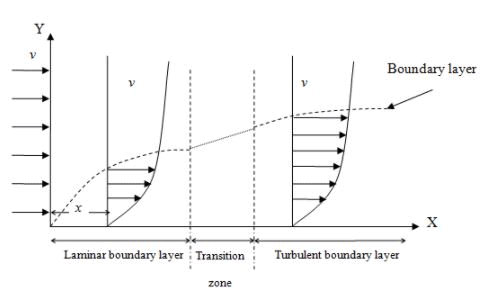Fig.4.1. Boundary layer flow past a flat plate

The velocity profile merges smoothly and asymptotically in the free shear and the boundary layer thickness is difficult to measure. However, the theoretical laminar boundary layer thickness can be calculated using the eq.4.1.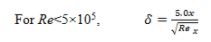(4.1)

where, Rex is the local Reynold number at a distance x. The derivative of δ can be found in any stated boundary.
The turbulent boundary layer thickness can be calculated using eq.4.2.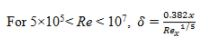(4.2)

Figure 4.2 shows the velocity boundary layer for the turbulent and laminar zone.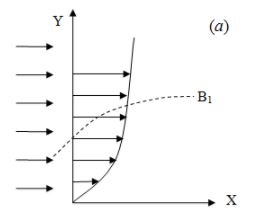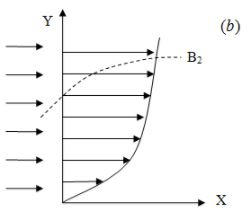Fig.4.2: Boundary layer flow past a flat surface (a) turbulent, and (b) laminar

In continuation to the above discussion and understanding, if the solid surface is maintained at a temperature, T, which is different from the fluid temperature, T, measured at a point far away from the surface, a variation of the temperature of the fluid is observed, which is similar to the velocity variation described. That is, the fluid temperature varies from Tat the wall toTf ­ far away from the wall, with most of the variation occurring close to the surface.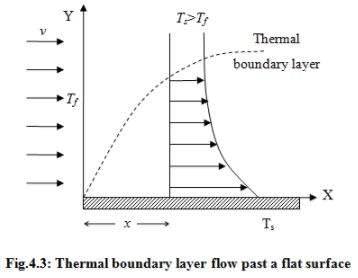If Ts >Tf , the fluid temperature approaches asymptotically and the temperature profile at a distance x is shown in fig.4.3. However, a thermal boundary may be defined (similar to velocity boundary) as the distance from the surface to the point where the temperature is within 1% of the free stream fluid temperature (T). Outside the thermal boundary layer the fluid is assumed to be a heat sink at a uniform temperature of T. The thermal boundary layer is generally not coincident with the velocity boundary layer, although it is certainly dependant on it. That is, the velocity, boundary layer thickness, the variation of velocity, whether the flow is laminar or turbulent etc are all the factors which determine the temperature variation in the thermal boundary layer. The thermal boundary layer and velocity boundary layer are related by the Prandtl number,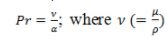is called the momentum diffusivity and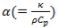is called the thermal diffusivity; is less than unity, the momentum boundary layer (or velocity boundary layer) remains within the thermal boundary layer. If Pr >1, the boundary layers will be reversed as shown in the fig.4.4. The thermal boundary layer and velocity boundary layer coincides at Pr =1.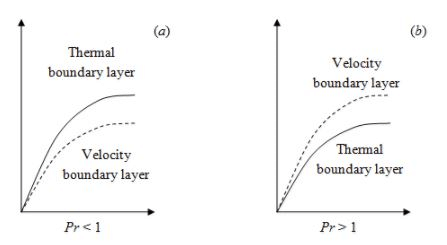Fig.4.4: The relation of two boundary layers at different Pr numbers

The above boundary layer theory will be helpful to understand the heat transfer in the process. Through the boundary layers heat transfer is covered in a separate chapter, but the detailed derivation and development of all the relationships having engineering importance for the prediction of forced convection heat transfer coefficient is beyond the scope of the course. The reader may consult any standard fluid mechanics and heat transfer books for detailed knowledge.

The purpose of this chapter is to present a collection of the most useful of the existing relations for the most frequently encountered cases of forced convection. Some of these relations will be having theoretical bases, and some will be empirical dimensionless correlations of experimental data. In some situations, more than one relation will be given.

The document Forced Convective Heat Transfer - 1 Notes | Study Heat Transfer - Mechanical Engineering is a part of the Mechanical Engineering Course Heat Transfer.
All you need of Mechanical Engineering at this link: Mechanical Engineering

## Heat Transfer

58 videos|70 docs|85 tests
 Use Code STAYHOME200 and get INR 200 additional OFF

## Heat Transfer

58 videos|70 docs|85 tests

Track your progress, build streaks, highlight & save important lessons and more!

,

,

,

,

,

,

,

,

,

,

,

,

,

,

,

,

,

,

,

,

,

;